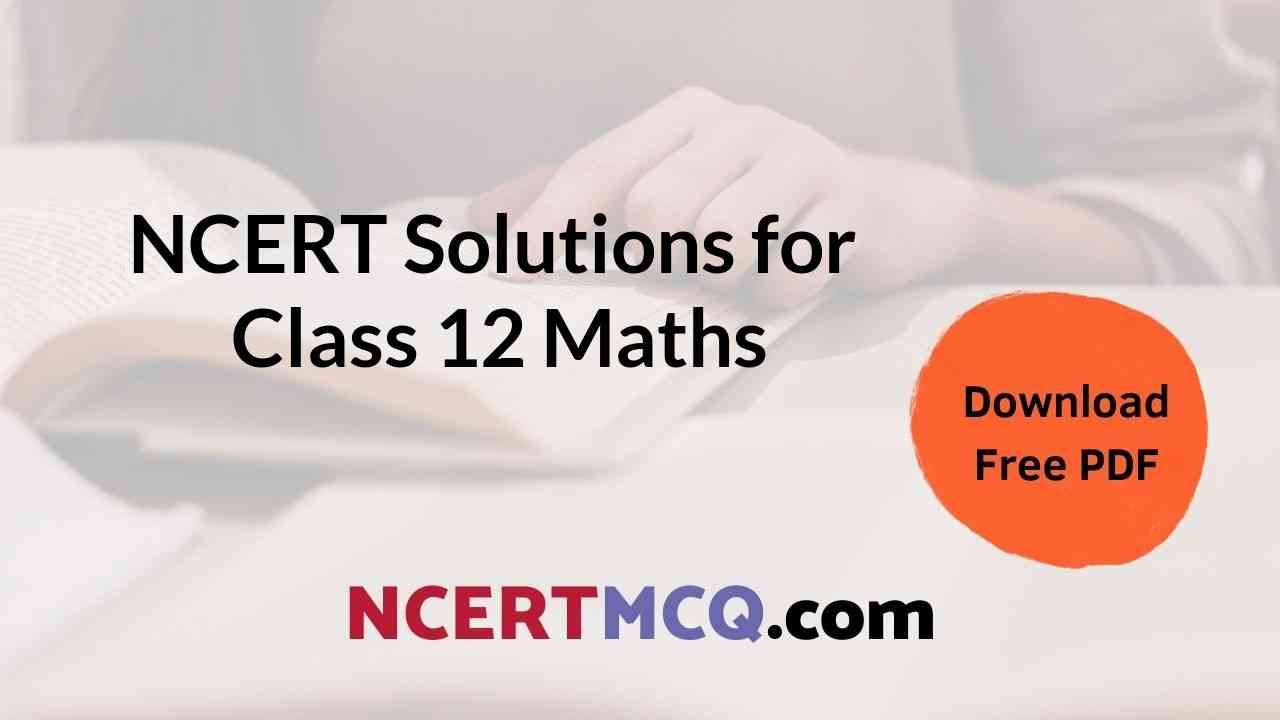## Class 12th Maths NCERT Solutions | NCERT Solutions of Maths Class 12

Maths NCERT Solutions Class 12 | Maths Class 12 NCERT Solutions

CBSE Class 12th Maths NCERT Solutions Chapter 1 Relations and Functions

NCERT 12 Maths Solutions Chapter 2 Inverse Trigonometric Functions

Solution of Maths Class 12 Chapter 3 Matrices

NCERT Maths Solution Class 12 Chapter 4 Determinants

12th CBSE Maths Solutions Chapter 5 Continuity and Differentiability

NCERT Maths Class 12 Solutions Chapter 6 Application of Derivatives

NCERT Solutions Maths Class 12 Chapter 7 Integrals

NCERT Solutions of Class 12 Maths Chapter 8 Application of Integrals

NCERT Solution of Maths Class 12 Chapter 9 Differential Equations

Class 12 Maths Solutions Chapter 10 Vector Algebra

Maths Class 12 NCERT Solutions Chapter 11 Three Dimensional Geometry

NCERT Class 12 Maths Solutions Chapter 12 Linear Programming

Maths NCERT Solutions Class 12 Chapter 13 Probability